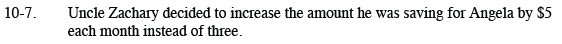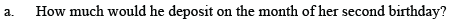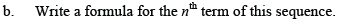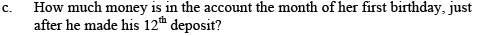Home > CCA2 > Chapter 10 > Lesson 10.1.1 > Problem10-7

10-7.
1. Uncle Zachary decided to increase the amount he was saving for Angela by $5 each month instead of three. 1. How much would he deposit on the month of her second birthday? Homework Help ✎ 2. Write a formula for the nth term of this sequence. 3. How much money is in the account the month of her first birthday, just after he made his 12th deposit?Recall that Uncle Zachary puts$50 in Angela's account the first month.
In this case he will put $55 in Angela's account the second month and$60 the third month.

The sequence is 50, 55, 60, 65, ..., which is linear. The equation should be of the form an + b.t(n) = 50 +5(n − 1) or t(n) = 5n + 45Add the first 12 terms of your sequence.